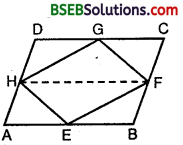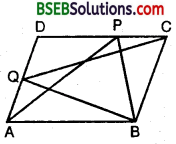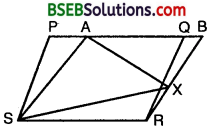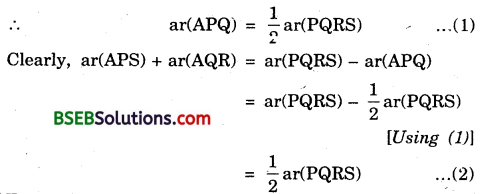# HSSlive: Plus One & Plus Two Notes & Solutions for Kerala State Board

## BSEB Class 9 Maths Chapter 9 Areas of Parallelograms and Triangles Ex 9.2 Textbook Solutions PDF: Download Bihar Board STD 9th Maths Chapter 9 Areas of Parallelograms and Triangles Ex 9.2 Book AnswersBSEB Class 9 Maths Chapter 9 Areas of Parallelograms and Triangles Ex 9.2 Textbook Solutions PDF: Download Bihar Board STD 9th Maths Chapter 9 Areas of Parallelograms and Triangles Ex 9.2 Book Answers

BSEB Class 9th Maths Chapter 9 Areas of Parallelograms and Triangles Ex 9.2 Textbooks Solutions and answers for students are now available in pdf format. Bihar Board Class 9th Maths Chapter 9 Areas of Parallelograms and Triangles Ex 9.2 Book answers and solutions are one of the most important study materials for any student. The Bihar Board Class 9th Maths Chapter 9 Areas of Parallelograms and Triangles Ex 9.2 books are published by the Bihar Board Publishers. These Bihar Board Class 9th Maths Chapter 9 Areas of Parallelograms and Triangles Ex 9.2 textbooks are prepared by a group of expert faculty members. Students can download these BSEB STD 9th Maths Chapter 9 Areas of Parallelograms and Triangles Ex 9.2 book solutions pdf online from this page.

## Bihar Board Class 9th Maths Chapter 9 Areas of Parallelograms and Triangles Ex 9.2 Books Solutions

 Board BSEB Materials Textbook Solutions/Guide Format DOC/PDF Class 9th Subject Maths Chapter 9 Areas of Parallelograms and Triangles Ex 9.2 Chapters All Provider Hsslive

## How to download Bihar Board Class 9th Maths Chapter 9 Areas of Parallelograms and Triangles Ex 9.2 Textbook Solutions Answers PDF Online?

2. Click on the Bihar Board Class 9th Maths Chapter 9 Areas of Parallelograms and Triangles Ex 9.2 Answers.
3. Look for your Bihar Board STD 9th Maths Chapter 9 Areas of Parallelograms and Triangles Ex 9.2 Textbooks PDF.
4. Now download or read the Bihar Board Class 9th Maths Chapter 9 Areas of Parallelograms and Triangles Ex 9.2 Textbook Solutions for PDF Free.

## BSEB Class 9th Maths Chapter 9 Areas of Parallelograms and Triangles Ex 9.2 Textbooks Solutions with Answer PDF Download

Find below the list of all BSEB Class 9th Maths Chapter 9 Areas of Parallelograms and Triangles Ex 9.2 Textbook Solutions for PDF’s for you to download and prepare for the upcoming exams:

## BSEB Bihar Board Class 9th Maths Solutions Chapter 9 Areas of Parallelograms and Triangles Ex 9.2

Question 1.
In figure, ABCD is a parallelogram, AE ⊥ DC and CF ⊥ AD. If AB = 16 cm, AE = 8 cm and CF = 10 cm, find AD.Solution:
We have,
Area of a ||gm = Base x Height
∴ Area of ||gm ABCD = AB x AE
= (16 x 8) cm² = 128 cm² … (1)
Also, Area of ||gm ABCD = AD x CF
I = (AD x 10) cm² … (2)
From (1) and (2), we get
⇒ AD = 12810 cm = 12.8 cm.

Question 2.
If E, F, G and H are respectively the mid-points of the sides of a parallelogram ABCD, show that ar (EFGH) = 12ar(ABCD).
Solution:
A HGF and ||gm HDCF stand on the same base HF and lie between the same parallels HF and DC.∴ ar(HEF) = 12 ar(ABFH) … (1)
Similarly, ∆ HEF and ||gm ABFH stand on the same base HF and lie between the same parallels HF and AB.
∴ ar(HEF) = 12 ar(ABFH) … (2)
∴ Adding (1) and (2), we get
ar(HGF) + ar(∆ HEF) = 12 [ar(HDCF) + ar(ABFH)]
⇒ ar(EFGH) = 12 ar(ABCD).

Question 3.
P and Q are any two points lying on the sides DC and AD respectively of a parallelogram ABCD. Show that ar(APB) = ar(BQC).
Solution:
∆ APB and ||gm ABCD stand on the same base AB and lie between the same parallels AB and DC.∴ ar(APB) = 12 ar(ABCD) … (1)
Similarly, ∆ BQC and ||gm ABCD stand on the same base BO and lie between the same parallels BC and AD.
∴ ar(BQC) = 12 ar(ABCD)
From (1) and (2), we have
ar(APB) = ar(BQC).

Question 4.
In figure, P is a point in the interior of a parallelogram ABCD. Show that
(i) ar(APB) + ar(PCD) = 12 ar(ABCD)
(ii) ar(APD) + ar(PBC) = ar(APB) + ar(PCD).Solution:
Draw EPF parallel to AB or DC and GPH parallel to AD or BC.Now AGHD is a ||gm [∵ GH || DA and AG || DH]
Similarly, HCBG, EFCD and ABFE are parallelograms.
(i) ∆ APB and ||gm ABFE stand on the same base AB and lie between the same parallels AB and DC.
∴ ar(APB) = 12 ar(ABFE) … (1)
Similarly, ar(PCD) = 12 ar(EFCD)
Adding (1) and (2), we get
ar(APB) + ar(PCD) = 12 [ar(ABFE) + ar(EFCD)]
= 12 ar(ABCD) … (3)

(ii) ∆ APD and ||gm AGHD are on the same base AD and lie between the same parallels AD and HG.
∴ ar(APD) = 12 ar(AGHD) … (4)
Similarly, ar(PCB) = 12ar(GBCH) … (5)
Adding (4) and (5), we get
ar(APD) + ar(PCB) = 12 [ar(AGHD) + ar(GBCH)]
= 12 ar(ABCD) … (6)
From (3) and (6), we get
ar(APD) + ar(PBC) = ar(APB) + ar(PCD).

Question 5.
In figure, PQRS and ABRS are parallelograms and X is any point on side BR. Show that
(i) ar(PQRS) = 12 ar(ABJtS)
(ii) ar(AXS) = 12 ar(PQRS).Solution:
(i) ||gm PQRS and ||gm ABRS stand on the same base RS and lie between the same parallels SR and PAQB.
∴ ar(PQRS) = ar(ABRS) … (1)

(ii) ∆ AXS and ||gm ABRS stand on the same base AS and lie between the same parallels AS and RB.
∴ ar(AXS) = 12 ar(ABRS) z
ar(AXS) = 12ar(PQRS) [Using (1)]

Question 6.
A farmer was having a field in the form of a parallelogram PQRS. She took any point A on RS and joined it to points P and Q. In how many parts the fields is divided? What are the shapes of these parts? The farmer wants to sow wheat and pulses in equal portions of the field separately. How should she do it?
Solution:
Clearly, the field i.e., ||gm PQRS is divided into 3 parts.Each part is of the shape of triangle.
Since ∆ APQ and ||gm PQRS stand on the same base PQ and lie between the same parallels PQ and SR.From (1) and (2), we get
ar(APS) + ar(AQR) = ar(APQ).
Thus the farmer should sow wheat and pulses either as [(∆s APS and AQR) or ∆ APQ] or as [∆ APQ or (∆s APS and AQR].

## Bihar Board Class 9th Maths Chapter 9 Areas of Parallelograms and Triangles Ex 9.2 Textbooks for Exam Preparations

Bihar Board Class 9th Maths Chapter 9 Areas of Parallelograms and Triangles Ex 9.2 Textbook Solutions can be of great help in your Bihar Board Class 9th Maths Chapter 9 Areas of Parallelograms and Triangles Ex 9.2 exam preparation. The BSEB STD 9th Maths Chapter 9 Areas of Parallelograms and Triangles Ex 9.2 Textbooks study material, used with the English medium textbooks, can help you complete the entire Class 9th Maths Chapter 9 Areas of Parallelograms and Triangles Ex 9.2 Books State Board syllabus with maximum efficiency.

## FAQs Regarding Bihar Board Class 9th Maths Chapter 9 Areas of Parallelograms and Triangles Ex 9.2 Textbook Solutions

#### How to get BSEB Class 9th Maths Chapter 9 Areas of Parallelograms and Triangles Ex 9.2 Textbook Answers??

Students can download the Bihar Board Class 9 Maths Chapter 9 Areas of Parallelograms and Triangles Ex 9.2 Answers PDF from the links provided above.

#### Can we get a Bihar Board Book PDF for all Classes?

Yes you can get Bihar Board Text Book PDF for all classes using the links provided in the above article.

## Important Terms

Bihar Board Class 9th Maths Chapter 9 Areas of Parallelograms and Triangles Ex 9.2, BSEB Class 9th Maths Chapter 9 Areas of Parallelograms and Triangles Ex 9.2 Textbooks, Bihar Board Class 9th Maths Chapter 9 Areas of Parallelograms and Triangles Ex 9.2, Bihar Board Class 9th Maths Chapter 9 Areas of Parallelograms and Triangles Ex 9.2 Textbook solutions, BSEB Class 9th Maths Chapter 9 Areas of Parallelograms and Triangles Ex 9.2 Textbooks Solutions, Bihar Board STD 9th Maths Chapter 9 Areas of Parallelograms and Triangles Ex 9.2, BSEB STD 9th Maths Chapter 9 Areas of Parallelograms and Triangles Ex 9.2 Textbooks, Bihar Board STD 9th Maths Chapter 9 Areas of Parallelograms and Triangles Ex 9.2, Bihar Board STD 9th Maths Chapter 9 Areas of Parallelograms and Triangles Ex 9.2 Textbook solutions, BSEB STD 9th Maths Chapter 9 Areas of Parallelograms and Triangles Ex 9.2 Textbooks Solutions,
Share: